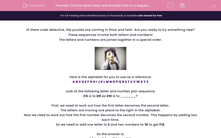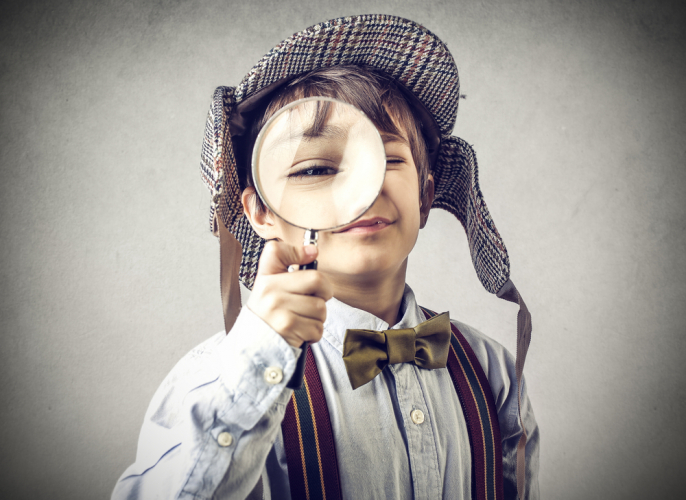# Find the Next Letter and Number Pair in a Sequence

In this worksheet, students will solve sequences involving combined letter and number pairs with more complex steps, involving addition and subtraction, and forward and reverse motion. It will develop students’ problem solving and sequencing skills.This content is premium and exclusive to EdPlace subscribers.Key stage:  KS 2

Curriculum topic:   Verbal Reasoning

Curriculum subtopic:   Letter Connections

Difficulty level:#### Worksheet Overview

Hi there code detective, the puzzles are coming in thick and fast!  Are you ready to try something new? These sequences involve both letters and numbers!

The letters and numbers are joined together in a special order.Here is the alphabet for you to use as a reference:

A B C D E F G H I J K L M N O P Q R S T U V W X Y Z

Look at the following letter and number pair sequence:

C6 is to D8 as E10 is to ______?

First, we need to work out how the first letter becomes the second letter.

The letters are moving one place to the right in the alphabet.

Now we need to work out how the first number becomes the second number. This happens by adding two each time.

So we need to add one letter to E and two numbers to 10 to get F12.

C6 is to D8 as E10 is to F12.Let’s try another:

G7 is to K9 as S12 is to ______?

First, we need to work out how the first letter becomes the second letter.

The letters are moving four places to the right in the alphabet.

Now we need to work out how the first number becomes the second number. This is adding two each time.

So we need to add four letters to S and two numbers to 12 to get W14.

G7 is to K9 as S12 is to W14.

In this activity, you will need to be sequence superstar to complete the number and letter pairs.